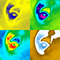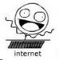# Don't you miss NArrays::set()?

Notice: This thread is very old.petr.pavel
Member | 533
+
0
-

Hi guys,
a couple of times I needed a way for setting an array item using an array of keys. A mirror feature to NArrays::get().

This is what I used:

``````/**
* Sets an array value, creating it if necessary.
* Example: \$val = NArrays::set(\$arr, array('i', 'j'), 123);
* @param  mixed  array
* @param  mixed  key
* @param  mixed  value
* @return mixed  value
*/
public static function set(array & \$arr, \$key, \$value)
{
\$_arr = & \$arr;
foreach (is_array(\$key) ? \$key : array(\$key) as \$k) {
\$_arr = & \$_arr[\$k];
}
return \$_arr = \$value;
}``````

It surprises me that nobody has needed it before. Am I missing something?Filip Procházka
Moderator | 4668
+
0
-

First of all, why the hell would you need function for this?

``\$arr['a']['b']['c'] = 'swag';``

second of all, there is one already

``````\$val =& Arrays::getRef(\$arr, ['a', 'b', 'c']);
\$val = 'swag';``````petr.pavel
Member | 533
+
0
-

Because the hell, I already have the keys in an array – and you don't know how many of them you've got (e.g. as a result of explode()). :-)

You're right about getRef(). Not very straight forward though.

Last edited by petr.pavel (2014-01-15 12:27)